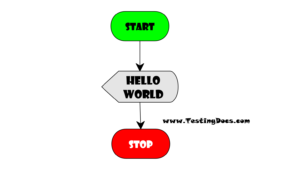Introduction The most basic program or the first flowchart to run is printing Hello World on the console. Let's build a simple flowchart to print "Hello World" on the console using RAPTOR tool. First Flowchart To create a RAPTOR program, you drag flowchart symbols from the left side to the ...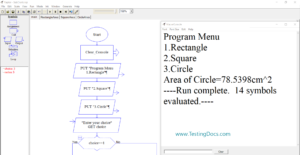Introduction In this tutorial, we will use the Raptor Subcharts flowchart to calculate the area of different shapes. The main chart of the Demo flowchart will prompt a program menu with options. Subcharts Demo The main flowchart has three options for Rectangle, Square, and Circle. Program Menu 1.Rectangle 2.Square 3.Circle ...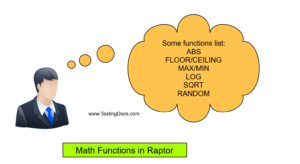Introduction In this tutorial, we will learn some mathematical functions that can be used in Raptor flowcharts with examples. Some of the mathematical functions are:
• MAX/MIN
• SQRT
• CEILING/FLOOR
• ABS
• LOG
MAX/MIN Max function returns the maximum of the two arguments supplied to the function. MIN function returns the ...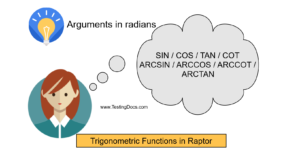Overview We can use trigonometric functions in Raptor flowcharts. Some of the functions are sin, cos, tan, arcsin, arccos, arctan, etc. The argument for the functions should be in radians. Let's look at the function usage with examples. sin sin(x) x should be in radians. cos cos(x) Sometimes we need ...Introduction Comments make RAPTOR flowcharts easy to understand by others. Its a good programming practice to add meaningful comments in to flowcharts. In this post, we will learn how to add comments to a RAPTOR flowcharts. Comments are ignored by the interpreter and compiler and are not executed. Comments are ...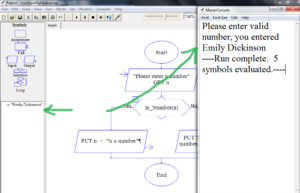Introduction In this article, we will learn how to perform user input validation in the Raptor flowchart. In this example, we will prompt the user to enter a number and we will display the number. It is a fairly easy example. But, the idea is to understand how do we ...